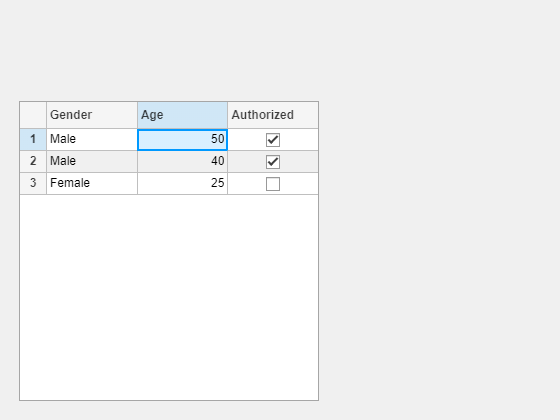# type

Class: matlab.uitest.TestCase
Package: matlab.uitest

Type in UI component

## Syntax

``type(testcase,comp,value)``
``type(testcase,uit,indices,value)``

## Description

example

````type(testcase,comp,value)` types `value` in the UI component `comp`.```

example

````type(testcase,uit,indices,value)` types `value` in the cell specified by `indices` within the table UI component `uit`.```

## Input Arguments

expand all

Instance of the test case, specified as a `matlab.uitest.TestCase` object.

Component to type in during test, specified as a UI component object that supports a type gesture. Components that support type gestures include edit fields and text areas.

Supported ComponentTypical Creation Function
Date Picker`uidatepicker`
Drop Down`uidropdown`
Edit Field (Numeric, Text)`uieditfield`
Spinner`uispinner`
Text Area`uitextarea`

Value to type into the component. The data type of `value` depends on the type of component under test. For example, if the component is a spinner, specify `value` as numeric. If the component is a text area or table, specify `value` as a character vector or string.

Target table UI component, specified as a `matlab.ui.control.Table` object. Table UI components are created with the `uitable` function.

Indices of the table cell to type in, specified as a 1-by-2 vector with the row index appearing before the column index.

Example: `[2 3]`

## Examples

expand all

Create a text edit field.

`ed = uieditfield('Value','Hello')`

Create an interactive test case and verify the initial value.

```tc = matlab.uitest.TestCase.forInteractiveUse; tc.verifyEqual(ed.Value,'Hello')```
`Verification passed.`

Type the word "Goodbye" in the edit field and verify the new value.

```value = 'Goodbye'; tc.type(ed,value) tc.verifyEqual(ed.Value,value)```
`Verification passed.`

Create an editable drop-down list.

`dropdown = uidropdown('Editable','on');`

Create an interactive test case and add a custom item to the drop-down list.

```tc = matlab.uitest.TestCase.forInteractiveUse; tc.type(dropdown,'Custom Item')```

Verify the new value.

`tc.verifyEqual(dropdown.Value,'Custom Item')`
`Verification passed.`

Create a table UI component that contains a mixture of different data types. Set the `ColumnEditable` property to `true` so that users can edit the data in the table.

```fig = uifigure; uit = uitable(fig); d = {'Male',52,true;'Male',40,true;'Female',25,false}; uit.Data = d; uit.ColumnName = {'Gender','Age','Authorized'}; uit.ColumnEditable = true;```

Create an interactive test case and verify the initial value of the table cell with indices (1,2).

```tc = matlab.uitest.TestCase.forInteractiveUse; tc.verifyEqual(uit.Data(1,2),{})```
```Verification passed. ```

Change the value of the cell to 50 and verify the new value.

`tc.type(uit,[1 2],'50')``tc.verifyEqual(uit.Data(1,2),{})`
```Verification passed. ```

## Version History

Introduced in R2018a

expand all